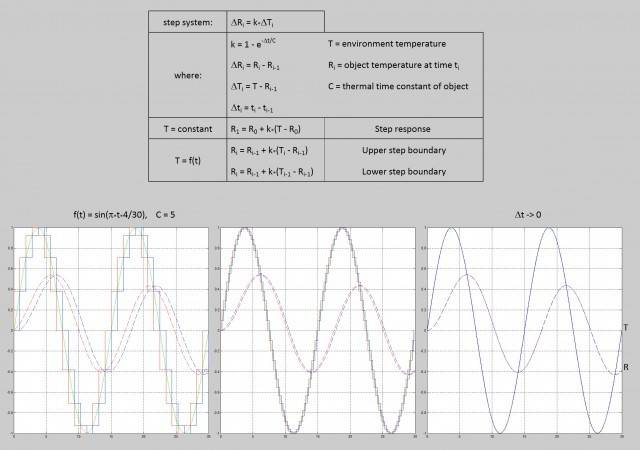# Transform difference equation into continuous function?

## Homework Statement

My question: Can I turn this difference equation for R below, into a continuous function R(t)? I have no idea if, or how, I can. And I'd like to.

Equation derived from the following manufacturer statement on the thermal response of a thermistor to a fixed temperature:

The time constant is the time in seconds required for the thermistor to change through 63.2 % of the difference between its initial and final body temperatures, when subjected to a step change in temperature

Using a small delta_t, relative to the time constant, the response of the thermistor to a constantly changing temperature T(t) was approximated to a continuous curve, via upper and lower step response boundaries, then graphed, which I have included with the rest of my work below.

## Homework Equations3. The Attempt at a Solution [/B]
I don't know how to attempt this. As I said, I'd like to be able to get the continuous function R(t) for a given T = f(t). Thanks.

haruspex
Homework Helper
Gold Member
2020 Award
If I am interpreting this aright, the underlying process is continuous (specifically, the difference between the object temperature and the environmental temperature declines negative exponentially). The discreteness only arises from the sampling intervals.
So, yes, you can pretend that the sampling is continuous to get a continuous equation relating dR/dt, R and T.

I realise I can pretend so to speak, the graphs illustrate that.

I want the actual continuous function though (not because I need it, but because I'm curious), and I'm having a brain lapse. I was hoping someone could point me in the direction of what to look up.

I am basically looking to do the opposite of what a computer programmer does to functions.

haruspex
Homework Helper
Gold Member
2020 Award
I realise I can pretend so to speak, the graphs illustrate that.

I want the actual continuous function though (not because I need it, but because I'm curious), and I'm having a brain lapse. I was hoping someone could point me in the direction of what to look up.

I am basically looking to do the opposite of what a computer programmer does to functions.
Not sure you understood my post, or maybe I still do not understand what you are trying to do.

It's fairly straightforward from the equations given. Replacing the differential operator Δ by the infinitesimal d:
##k=1-e^{-dt/C} \rightarrow dt/C##, so ##dR=(dt/C)(T-R)##, hence ##C\dot R=T-R##. In other words, R tends towards the current value of T at a rate proportional to the difference from that value. Exactly as you would expect, no?

In general, T is a function of t. If given the function, you may be able to solve the differential equation. Looks like you are interested in the specific case where T is a sine function of t, ##C\dot R= A\sin(\omega t)-R##. Can you solve that?

•Archie Medes
Thank you for the help, I'm mostly there, I can now solve R(t) for a given T(t). I was not interested in that sine function per se, it was just a simple bounded continuously changing function that I could use for R to follow on a graph.

What I'm still unclear about, is this part:
##k=1-e^{-dt/C} \rightarrow dt/C##
How did k become just dt/C?

Something is clearly lacking from my fundamental understanding of the topic

haruspex
Homework Helper
Gold Member
2020 Award
How did k become just dt/C?
ex≈1+x+x2/2+...., so k=1-e-dt/C≈1-(1-dt/C+(dt/C)2/2-....)=dt/C-....
Dividing through by dt and taking the limit as dt→0, all but the 1/C term disappears.

But really the question is the other way about. How did dt/C turn into 1-e-dt/C?
If we do not know what T(t) is, except by taking samples at intervals, then in order to predict R we need to make some assumption about what T was doing between the samples. If you suppose it is constant, only changing in jumps at the interval boundaries, and solve ##C\dot R=T-R## for one interval, I believe you will get the negative exponential form. Maybe a better assumption would be that T is linear in between, or some other fit to the datapoints, but the equation might be harder to solve.

•Archie Medes
Ahh, thank you

Looks like the thing lacking from my understanding in this case is the derivation of series expansions of functions. Right now is the first time I have read the formal definition of the Taylor expansion of a function. I have used expansions to solve problems, and I'm fine using sigma notation, but I still don't understand the derivations. But now I have an idea what I need to study.

But really the question is the other way about. How did dt/C turn into 1-e-dt/C?
Heh, I just pulled 1-e-1 out of the recesses of my memory when I read 63.2% in the definition of the thermistor response to a step change in temperature. I agree that using a step change is not ideal, but that was the definition, so I used a maximum and minimum step, which could be used to compute somewhere closer to the true point in between. In a real sampled environment, with a constantly changing temperature, I could eliminate k altogether. It would only fail if delta_R and delta_T readings became too small to be meaningful. This is a purely mathematical curiosity. I get frustrated when I don't understand things.

Once again, thank you, you've been a big help :)